# Resources tagged with: Area - triangles, quadrilaterals, compound shapes

Filter by: Content type:
Age range:
Challenge level:

There are 53 NRICH Mathematical resources connected to Area - triangles, quadrilaterals, compound shapes, you may find related items under Measuring and calculating with units.

Broad Topics > Measuring and calculating with units > Area - triangles, quadrilaterals, compound shapes### Isometric Areas

##### Age 11 to 14Challenge Level

We usually use squares to measure area, but what if we use triangles instead?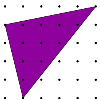### Triangles in a Square

##### Age 11 to 14Challenge Level

What are the possible areas of triangles drawn in a square?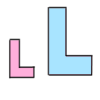### Growing Rectangles

##### Age 11 to 14Challenge Level

What happens to the area and volume of 2D and 3D shapes when you enlarge them?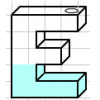### Maths Filler

##### Age 11 to 14Challenge Level

Imagine different shaped vessels being filled. Can you work out what the graphs of the water level should look like?### Gutter

##### Age 14 to 16Challenge Level

Manufacturers need to minimise the amount of material used to make their product. What is the best cross-section for a gutter?### Trapezium Four

##### Age 14 to 16Challenge Level

The diagonals of a trapezium divide it into four parts. Can you create a trapezium where three of those parts are equal in area?### Areas of Parallelograms

##### Age 14 to 16Challenge Level

Can you find the area of a parallelogram defined by two vectors?### Isosceles Triangles

##### Age 11 to 14Challenge Level

Draw some isosceles triangles with an area of $9$cm$^2$ and a vertex at (20,20). If all the vertices must have whole number coordinates, how many is it possible to draw?### Tilted Squares

##### Age 11 to 14Challenge Level

It's easy to work out the areas of most squares that we meet, but what if they were tilted?### Inscribed in a Circle

##### Age 14 to 16Challenge Level

The area of a square inscribed in a circle with a unit radius is, satisfyingly, 2. What is the area of a regular hexagon inscribed in a circle with a unit radius?### Pick's Theorem

##### Age 14 to 16Challenge Level

Polygons drawn on square dotty paper have dots on their perimeter (p) and often internal (i) ones as well. Find a relationship between p, i and the area of the polygons.### Of All the Areas

##### Age 14 to 16Challenge Level

Can you find a general rule for finding the areas of equilateral triangles drawn on an isometric grid?##### Age 14 to 18Challenge Level##### Age 14 to 16Challenge Level

In this problem we are faced with an apparently easy area problem, but it has gone horribly wrong! What happened?### So Big

##### Age 16 to 18Challenge Level

One side of a triangle is divided into segments of length a and b by the inscribed circle, with radius r. Prove that the area is: abr(a+b)/ab-r^2### Areas and Ratios

##### Age 16 to 18Challenge Level

Do you have enough information to work out the area of the shaded quadrilateral?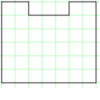### Rod Area

##### Age 7 to 11

This task challenges you to create symmetrical U shapes out of rods and find their areas.### Triangle Transformation

##### Age 7 to 14Challenge Level

Start with a triangle. Can you cut it up to make a rectangle?### Another Triangle in a Triangle

##### Age 16 to 18Challenge Level

Can you work out the fraction of the original triangle that is covered by the green triangle?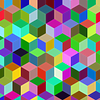### More Isometric Areas

##### Age 11 to 14Challenge Level

Isometric Areas explored areas of parallelograms in triangular units. Here we explore areas of triangles...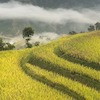### The Farmers' Field Boundary

##### Age 11 to 14Challenge Level

The farmers want to redraw their field boundary but keep the area the same. Can you advise them?### Triangle in a Trapezium

##### Age 11 to 16Challenge Level

Can you find and prove the relationship between the area of a trapezium and the area of a triangle constructed within it?### Towering Trapeziums

##### Age 14 to 16Challenge Level

Can you find the areas of the trapezia in this sequence?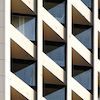##### Age 11 to 14Challenge Level

We started drawing some quadrilaterals - can you complete them?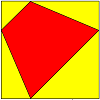##### Age 11 to 14Challenge Level### Kite in a Square

##### Age 14 to 18Challenge Level

Can you make sense of the three methods to work out what fraction of the total area is shaded?##### Age 14 to 16Challenge Level

Investigate the properties of quadrilaterals which can be drawn with a circle just touching each side and another circle just touching each vertex.### Maths Filler 2

##### Age 14 to 16Challenge Level

Can you draw the height-time chart as this complicated vessel fills with water?### Uncanny Triangles

##### Age 7 to 11Challenge Level

Can you help the children find the two triangles which have the lengths of two sides numerically equal to their areas?### Shear Magic

##### Age 11 to 14Challenge Level

Explore the area of families of parallelograms and triangles. Can you find rules to work out the areas?### Dotty Triangles

##### Age 11 to 14Challenge Level

Imagine an infinitely large sheet of square dotty paper on which you can draw triangles of any size you wish (providing each vertex is on a dot). What areas is it/is it not possible to draw?### Biggest Enclosure

##### Age 14 to 16Challenge Level

Three fences of different lengths form three sides of an enclosure. What arrangement maximises the area?### Squ-areas

##### Age 14 to 16Challenge Level

Three squares are drawn on the sides of a triangle ABC. Their areas are respectively 18 000, 20 000 and 26 000 square centimetres. If the outer vertices of the squares are joined, three more. . . .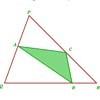### Halving the Triangle

##### Age 16 to 18Challenge Level

Draw any triangle PQR. Find points A, B and C, one on each side of the triangle, such that the area of triangle ABC is a given fraction of the area of triangle PQR.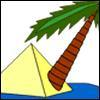### Triangle Island

##### Age 7 to 11Challenge Level

You have pitched your tent (the red triangle) on an island. Can you move it to the position shown by the purple triangle making sure you obey the rules?### Rati-o

##### Age 11 to 14Challenge Level

Points P, Q, R and S each divide the sides AB, BC, CD and DA respectively in the ratio of 2 : 1. Join the points. What is the area of the parallelogram PQRS in relation to the original rectangle?### Disappearing Square

##### Age 11 to 14Challenge Level

Do you know how to find the area of a triangle? You can count the squares. What happens if we turn the triangle on end? Press the button and see. Try counting the number of units in the triangle now. . . .### Overlap

##### Age 14 to 16Challenge Level

A red square and a blue square overlap. Is the area of the overlap always the same?### Square Pizza

##### Age 14 to 16Challenge Level

Can you show that you can share a square pizza equally between two people by cutting it four times using vertical, horizontal and diagonal cuts through any point inside the square?### Rhombus in Rectangle

##### Age 14 to 16Challenge Level

Take any rectangle ABCD such that AB > BC. The point P is on AB and Q is on CD. Show that there is exactly one position of P and Q such that APCQ is a rhombus.##### Age 11 to 14Challenge Level

Four rods, two of length a and two of length b, are linked to form a kite. The linkage is moveable so that the angles change. What is the maximum area of the kite?### Isosceles

##### Age 11 to 14Challenge Level

Prove that a triangle with sides of length 5, 5 and 6 has the same area as a triangle with sides of length 5, 5 and 8. Find other pairs of non-congruent isosceles triangles which have equal areas.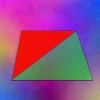### Same Height

##### Age 14 to 16Challenge Level

A trapezium is divided into four triangles by its diagonals. Can you work out the area of the trapezium?##### Age 14 to 16Challenge Level

The points P, Q, R and S are the midpoints of the edges of a non-convex quadrilateral.What do you notice about the quadrilateral PQRS and its area?### From All Corners

##### Age 14 to 16Challenge Level

Straight lines are drawn from each corner of a square to the mid points of the opposite sides. Express the area of the octagon that is formed at the centre as a fraction of the area of the square.### Kissing Triangles

##### Age 11 to 14Challenge Level

Determine the total shaded area of the 'kissing triangles'.### Dividing the Field

##### Age 14 to 16Challenge Level

A farmer has a field which is the shape of a trapezium as illustrated below. To increase his profits he wishes to grow two different crops. To do this he would like to divide the field into two. . . .### Equilateral Areas

##### Age 14 to 16Challenge Level

ABC and DEF are equilateral triangles of side 3 and 4 respectively. Construct an equilateral triangle whose area is the sum of the area of ABC and DEF.### Diagonals for Area

##### Age 16 to 18Challenge Level

Can you prove this formula for finding the area of a quadrilateral from its diagonals?### Golden Triangle

##### Age 16 to 18Challenge Level

Three triangles ABC, CBD and ABD (where D is a point on AC) are all isosceles. Find all the angles. Prove that the ratio of AB to BC is equal to the golden ratio.